# 机器学习技法笔记(5)-Soft Margin SVM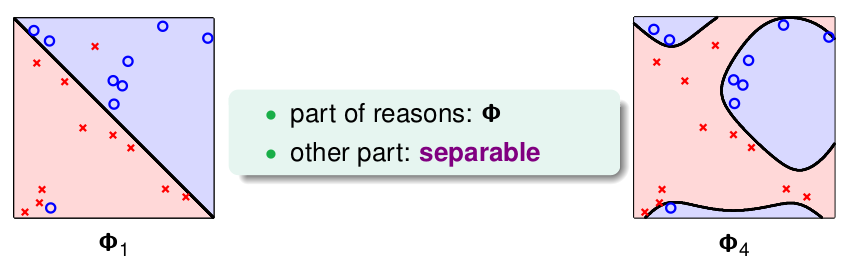Pocket找的是分类错误点尽可能少的分类面，不一定必须完全分开，容许错误的发生。而我们之前的SVM均是要求所有的数据都必须分类正确，如果我们将Pocket与SVM结合，即允许发生错误，如下: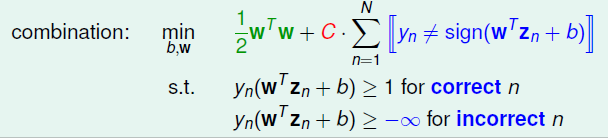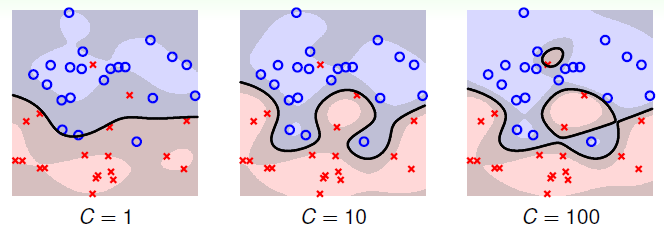\begin{align} \min\limits_{b,\mathbf{w},\xi} & \quad \frac{1}{2}\mathbf{w}^T\mathbf{w}+C \cdot \sum\limits_{n=1}^N \xi_n \\ s.t. & \quad y_n(\mathbf{w}^T{\mathbf{z}}_n+b) \geq 1 - \xi_n, for \ all \ n; \\ & \quad \xi_n \geq 0, for \ all \ n\end{align}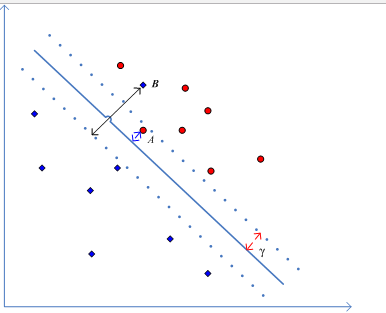\begin{align}\mathcal{L}(b, \mathbf{w}, \xi, \alpha, \beta) = &\frac{1}{2}\mathbf{w}^T\mathbf{w}+C \cdot \sum\limits_{n=1}^N \xi_n \\&+ \sum\limits_{n=1}^{N}\alpha_n(1-\xi_n-y_n(\mathbf{w}^T\mathbf{z}_n+b)) \\ & +\sum\limits_{n=1}^{N}\beta_n(-\xi_n) \end{align}

$\max\limits_{\alpha_n \geq 0,\ \beta \geq 0}\left(\min\limits_{b, \mathbf{w}, \xi} \quad \mathcal{L(b, \mathbf{w}, \xi, \alpha, \beta)}\right)$

$\max\limits_{ 0 \leq \alpha_n \leq C}\left(\min\limits_{b, \mathbf{w}} \quad \frac{1}{2}\mathbf{w}^T{\mathbf{w}} + \sum\limits_{n=1}^{N}\alpha_n(1-y_n(\mathbf{w}^T\mathbf{z}+b))\right)$

\begin{align} \min\limits_{\alpha} & \quad \frac{1}{2}\sum\limits_{n=1}^N\sum\limits_{m=1}^N \alpha_n\alpha_my_ny_m\mathbf{z}_n^T\mathbf{z}_m - \sum\limits_{n=1}^{N}\alpha_n \\ s.t. & \quad \sum\limits_{n=1}^{N}\alpha_ny_n = 0; \\ & \quad 0 \leq \alpha_n \leq C, \ n = 1,2...N \end{align}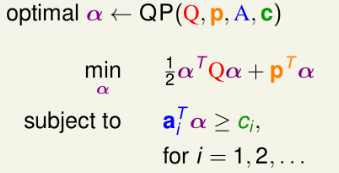• Q矩阵元素: $q_{n,m} = y_ny_m\mathbf{z}_n^T\mathbf{z}_m$
• $p = -_N$
• 对于$A,c$，对应如下:
• $a_{\geq} = y, c_{\geq} = 0$
• $a_{\leq} = -y, c_{\leq} = 0$
• 对于$\alpha \geq 0$$a_n^T =$ n-th unit dircection, $c_n = 0$
• 对于$\alpha \leq C$$a_n^T =$ n-th unit direction, $c_n = -C$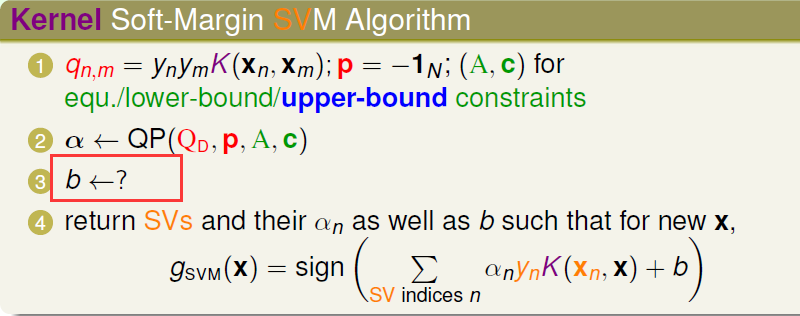\begin{align} \alpha_n(1-\xi_n-y_n(\mathbf{w}^T\mathbf{z}_n+b)) &=0 \\ \beta_n \xi_n = (C- \alpha_n)\xi_n & =0\end{align}

$b=y_s - \mathbf{w}^T\mathbf{z}_s = y_s - \sum\limits_{free \ SV} \alpha_ny_nK(\mathbf{x}_n, \mathbf{x}_s)$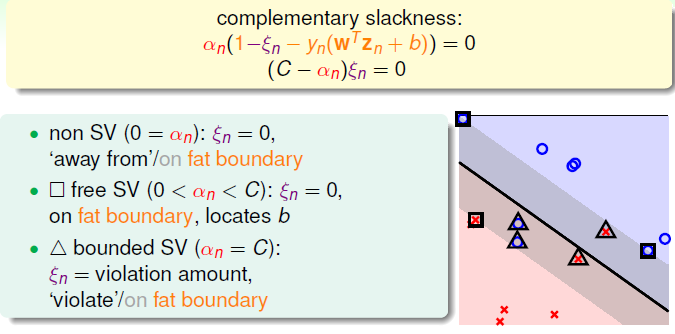• 非SV(支撑向量): 也就是距离分离面比较远的那些数据，如图中的圈圈叉叉，对应的$\alpha_n = 0, \xi_n = 0$
• free SV: 也就是说$\alpha_n < C, \xi_n =0$，它们在边界上, 满足条件。图中方框标记的点，我们使用这些点计算的$b$
• bounded SV: $\alpha_n =C, \xi_n > 0$，这种类型的数据，不满足margin条件，或者分错类别的，如图中三角标记的。